# High School Math : Right Triangles

## Example Questions

### Example Question #56 : How To Find The Length Of The Hypotenuse Of A Right Triangle : Pythagorean Theorem

The lengths of the sides of a triangle are consecutive odd numbers and the triangle's perimeter is 57 centimeters. What is the length, in centimeters, of its longest side?

23

21

25

17

19

21

Explanation:

First, define the sides of the triangle. Because the side lengths are consecutive odd numbers, if we define the shortest side will be as, the next side will be defined as, and the longest side will be defined as. We can then find the perimeter of a triangle using the following formula:Substitute in the known values and variables.Subtract 6 from both sides of the equation.Divide both sides of the equation by 3.Solve.This is not the answer; we need to find the length of the longest side, orSubstitute in the calculated value forand solve.The longest side of the triangle is 21 centimeters long.

### Example Question #21 : Right Triangles

Each of the following answer choices lists the side lengths of a different triangle.  Which of these triangles does not have a right angle?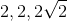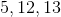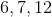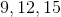Explanation:cannot be the side lengths of a right triangle.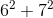does not equal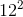. Also, special right triangle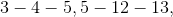and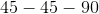rules can eliminate all the other choices.

### Example Question #1 : How To Find The Perimeter Of A Right Triangle

What is the perimeter of a triangle with side lengths of 5, 12, and 13?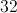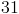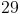Explanation:

To find the perimeter of a triangle you must add all of the side lengths together.

In this case our equation would look like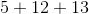Add the numbers together to get the answer.

### Example Question #2 : How To Find The Perimeter Of A Right Triangle

Three points in the xy-coordinate system form a triangle.

The points are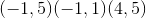.

What is the perimeter of the triangle?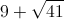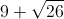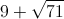Explanation:

Drawing points gives sides of a right triangle of 4, 5, and an unknown hypotenuse.

Using the pythagorean theorem we find that the hypotenuse is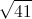.

### Example Question #3 : How To Find The Perimeter Of A Right Triangle

Find the perimeter of the following triangle: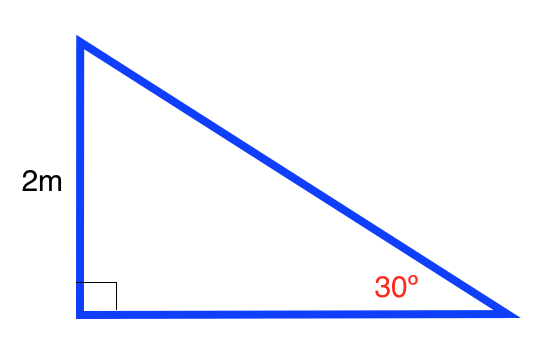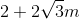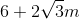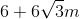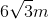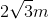Explanation:

The formula for the perimeter of a right triangle is: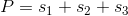whereis the length of a side.

Use the formulas for a a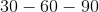triangle to find the length of the base. The formula for atriangle is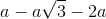.

Ourtriangle is: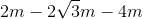Plugging in our values, we get: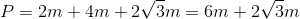### Example Question #4 : How To Find The Perimeter Of A Right Triangle

Find the perimeter of the following right triangle: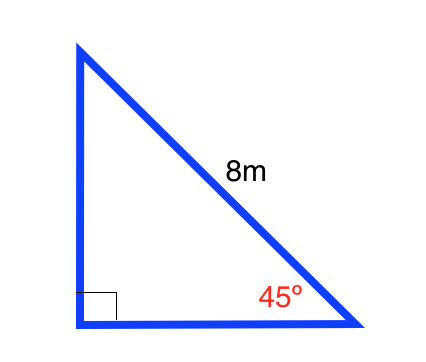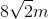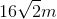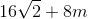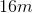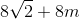Explanation:

The formula for the perimeter of a right triangle is:whereis the length of a side.

Use the formulas for a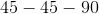triangle to find the length of the base and height. The formula for atriangle is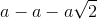Ourtriangle is: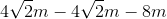Plugging in our values, we get: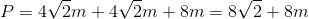### Example Question #5 : How To Find The Perimeter Of A Right Triangle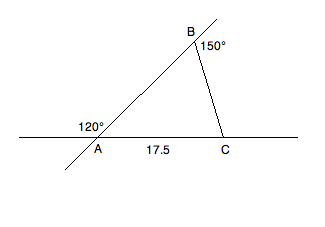Based on the information given above, what is the perimeter of triangle ABC?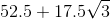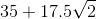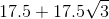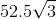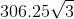Explanation: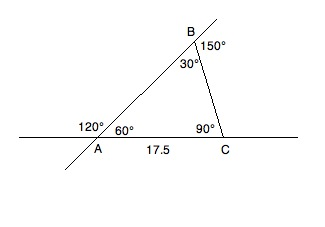Consult the diagram above while reading the solution. Because of what we know about supplementary angles, we can fill in the inner values of the triangle. Angles A and B can be found by the following reductions:

A + 120 = 180; A = 60

B + 150 = 180; B = 30

Since we know A + B + C = 180 and have the values of A and B, we know:

60 + 30 + C = 180; C = 90

This gives us a 30:60:90 triangle. Now, since 17.5 is across from the 30° angle, we know that the other two sides will have to be √3 and 2 times 17.5; therefore, our perimeter will be as follows: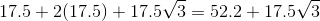### Example Question #10 : Triangles

A right triangle has a total perimeter of 12, and the length of its hypotenuse is 5. What is the area of this triangle?

10

6

12

3

15

6

Explanation:

The area of a triangle is denoted by the equation 1/2 b x h.

b stands for the length of the base, and h stands for the height.

Here we are told that the perimeter (total length of all three sides) is 12, and the hypotenuse (the side that is neither the height nor the base) is 5 units long.

So, 12-5 = 7 for the total perimeter of the base and height.

7 does not divide cleanly by two, but it does break down into 3 and 4,

and 1/2 (3x4) yields 6.

Another way to solve this would be if you recall your rules for right triangles, one of the very basic ones is the 3,4,5 triangle, which is exactly what we have here

### Example Question #1 : How To Find The Area Of A Right Triangle

The perimeter of a right triangle is 40 units. If the lengths of the sides are,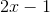, and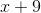units, then what is the area of the triangle?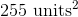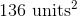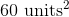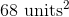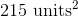Explanation:

Because the perimeter is equal to the sum of the lengths of the three sides of a triangle, we can add the three expressions for the lengths and set them equal to 40.

Perimeter: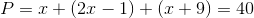Simplify the x terms.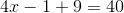Simplify the constants.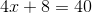Subtract 8 from both sides.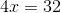Divide by 4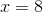One side is 8.

The second side is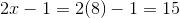.

The third side is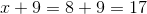.

Thus, the sides of the triangle are 8, 15, and 17.

The question asks us for the area of the triangle, which is given by the formula (1/2)bh. We are told it is a right triangle, so we can use one of the legs as the base, and the other leg as the height, since the legs will intersect at right angles. The legs of the right triangle must be the smallest sides (the longest must be the hypotenuse), which in this case are 8 and 15. So, let's assume that 8 is the base and 15 is the height.

The area of a triangle is (1/2)bh. We can substitute 8 and 15 for b and h.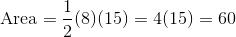.

The answer is 60 units squared.

### Example Question #7 : TrianglesFigure not drawn to scale.

In the figure above, rays PA and PB are tangent to circle O at points A and B, respectively. If the diameter of circle O is 16 units and the length of line segment PO is 17 units, what is the area, in square units, of the quadrilateral PAOB?

136

240

68

60

120

120

Explanation:

Because PA and PB are tangent to circle O, angles PAO and PBO must be right angles; therefore, triangles PAO and PBO are both right triangles.

Since AO and OB are both radii of circle O, they are congruent. Furthermore, because PA and PB are external tangents originating from the same point, they must also be congruent.

So, in triangles PAO and PBO, we have two sides that are congruent, and we have a congruent angle (all right angles are congruent) between them. Therefore, by the Side-Angle-Side (SAS) Theorem of congruency, triangles PAO and PBO are congruent.

Notice that quadrilateral PAOB can be broken up into triangles PAO and PBO. Since those triangles are congruent, each must comprise one half of the area of quadrilateral PAOB. As a result, if we find the area of one of the triangles, we can double it in order to find the area of the quadrilateral.

Let's determine the area of triangle PAO. We have already established that it is a right triangle. We are told that PO, which is the hypotenuse of the triangle, is equal to 17. We are also told that the diameter of circle O is 16, which means that every radius of the circle is 8, because a radius is half the size of a diameter. Since segment AO is a radius, its length must be 8.

So, triangle PAO is a right triangle with a hypotenuse of 17 and a leg of 8. We can use the Pythagorean Theorem in order to find the other leg. According to the Pythagorean Theorem, if a and b are the lengths of the legs of a right triangle, and c is the length of the hypotenuse, then:

a2 + b2 = c2

Let us let b represent the length of PA.

82 + b2 = 172

64 + b2 = 289

Subtract 64 from both sides.

b2 = 225

Take the square root of both sides.

b = 15

This means that the length of PA is 15.

Now let's apply the formula for the area of a right triangle. Because the legs of a right triangle are perpendicular, one can be considered the base, and the other can be considered the height of the triangle.

area of triangle PAO = (1/2)bh

= (1/2)(8)(15) = 60

Ultimately, we must find the area of quadrilateral PAOB; however, we previously determined that triangles PAO and PBO each comprise half of the quadrilateral. Thus, if we double the area of PAO, we would get the area of quadrilateral PAOB.

Area of PAOB = 2(area of PAO)

= 2(60) = 120 square units